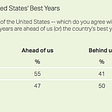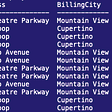# Map of the US“If there’s a place you gotta go I’m the one you need to know. I’m the map.”

## 1. As usual:

`import pandas as pdimport geopandasimport mathimport matplotlib.pyplot as plt`

## 2. Make sure to download all the files in the states_2020 folder

`states = geopandas.read_file('states_2020/cb_2020_us_state_5m.shp')states.head()`

## 3. PolygonPatch

`from descartes.patch import PolygonPatch`

## 4. Make a mini plot

`states.plot(); `

## 5. Get rid of excess states

`states_50 = states[~states['STUSPS'].isin(['GU', 'AS', 'AK', 'PR', 'VI', 'MP'])]states_50.head()`
`states_50.plot();`

## 6. Boundary plot

`states_50.boundary.plot(figsize=(40,15))`

## 7. Labeled US map

`fig, ax = plt.subplots(figsize=(29,12))states_50.apply(lambda x: ax.annotate(s=x.STUSPS, xy=x.geometry.centroid.coords, ha='center', fontsize=11, color = 'black'), axis=1);states_50.boundary.plot(ax=ax, color='black', linewidth = .2)states_50.plot(ax=ax, cmap = 'Blues')plt.savefig('US_state_map')`
`fig, ax = plt.subplots(figsize=(29,12))states_50.apply(lambda x: ax.annotate(s=x.STUSPS, xy=x.geometry.centroid.coords, ha='center', fontsize=11, color = 'white'), axis=1);states_50.boundary.plot(ax=ax, color='black', linewidth = .2)states_50.plot(ax=ax, cmap = 'flag')`

--

--

--

## More from Raizel Bernstein

Aspiring Data Scientist

Love podcasts or audiobooks? Learn on the go with our new app.

## A Streamlit dashboard: 5 steps from zero to Docker-deployed## 7 Best Github Repositories For Learning Data Science## Introducing Confidence Intervals## Data Science Fundamentals (R): Import & Export Data in Excel — xlsx## Instacart Market Basket Analysis Part 2: Which Groups of Customers Are Similar?## GDPR Implications for Data Science## EPISODE #15: Leveraging Predictive Aanalytics to Transform IT From Reactive to Proactive## Murray EMS names EMT and Paramedic of the Year The Daily Citizen## Raizel Bernstein

Aspiring Data Scientist

## Chicago Crime — A High Level Data Analysis## A visual comparison of COVID-19 Case Numbers and Vaccine Propagation in the US## Detailed Examples of SQLite WHERE Clause## Wine data set: A Classification Problem